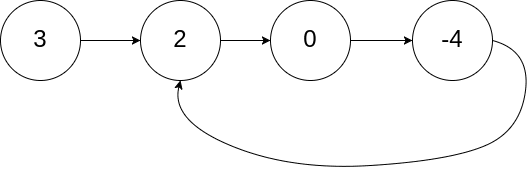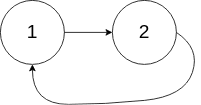# Question

Formatted question description: https://leetcode.ca/all/142.html

Given the head of a linked list, return the node where the cycle begins. If there is no cycle, return null.

There is a cycle in a linked list if there is some node in the list that can be reached again by continuously following the next pointer. Internally, pos is used to denote the index of the node that tail's next pointer is connected to (0-indexed). It is -1 if there is no cycle. Note that pos is not passed as a parameter.

Do not modify the linked list.

Example 1:Input: head = [3,2,0,-4], pos = 1
Output: tail connects to node index 1
Explanation: There is a cycle in the linked list, where tail connects to the second node.


Example 2:Input: head = [1,2], pos = 0
Output: tail connects to node index 0
Explanation: There is a cycle in the linked list, where tail connects to the first node.


Example 3:Input: head = , pos = -1
Output: no cycle
Explanation: There is no cycle in the linked list.


Constraints:

• The number of the nodes in the list is in the range [0, 104].
• -105 <= Node.val <= 105
• pos is -1 or a valid index in the linked-list.

Follow up: Can you solve it using O(1) (i.e. constant) memory?

# Algorithm

Fast and slow pointers, but this time you want to record where the two pointers meet. When the two pointers meet, let one of the pointers start from the head of the linked list, then start moving again, and the position where they meet again is the starting position of the ring in the linked list.

Because the fast pointer moves 2 each time and the slow pointer moves 1 each time, the fast pointer travels twice as far as the slow pointer. And the fast pointer goes one more circle than the slow pointer.

So the distance from head to the starting point of the ring, plus, the starting point of the ring to the point where they meet, is equal to the distance of one circle of the ring. Now start again, the distance from head to the starting point of the ring and the distance from the meeting point to the starting point of the ring is also equal, which is equivalent to subtracting the distance from the beginning of the ring to the point where they meet.

    a            b
A ------ B --------+
|         |
c |         |
C --------+

* A: 起始点
* B: Cycle Begins
* C: 1st 快慢指针相遇点

* A->B: a
* B->C: b
* C->B: c
* 环的长度 (b+c) 为 R



To understand this solution, you just need to ask yourself these question.

Assume the distance from head to the start of the loop is a the distance from the start of the loop to the point fast and slow meet is b the distance from the point fast and slow meet to the start of the loop is c

• What is the distance fast moved?
• What is the distance slow moved?
• And their relationship?

fast: a + b + c + b

slow: a + b

relationship - fast is 2X faster than slow: a + b + c + b = 2 * (a + b)

Thus a = c

# Code

• public class Linked_List_Cycle_II {

/**
* class ListNode {
*     int val;
*     ListNode next;
*     ListNode(int x) {
*         val = x;
*         next = null;
*     }
* }
*/
public class Solution {

/*
To understand this solution, you just need to ask yourself these question.

Assume the distance from head to the start of the loop is x1
the distance from the start of the loop to the point fast and slow meet is x2
the distance from the point fast and slow meet to the start of the loop is x3

What is the distance fast moved?
What is the distance slow moved?
And their relationship?

x1 + x2 + x3 + x2
x1 + x2
x1 + x2 + x3 + x2 = 2 (x1 + x2)

Thus x1 = x3

Finally got the idea.

*/

boolean circle = false;

while (fast != null && fast.next != null) {

fast = fast.next.next;
slow = slow.next;

// if (fast == slow)
if (fast == slow) {
circle = true;
break;
}
}

if (circle) {
while (fast != slow) {
fast = fast.next;
slow = slow.next;
}

return fast;
}

return null;

}
}

}

############

/**
* class ListNode {
*     int val;
*     ListNode next;
*     ListNode(int x) {
*         val = x;
*         next = null;
*     }
* }
*/
public class Solution {
boolean hasCycle = false;
while (!hasCycle && fast != null && fast.next != null) {
slow = slow.next;
fast = fast.next.next;
hasCycle = slow == fast;
}
if (!hasCycle) {
return null;
}
while (p != slow) {
p = p.next;
slow = slow.next;
}
return p;
}
}

• // OJ: https://leetcode.com/problems/linked-list-cycle-ii/
// Time: O(N)
// Space: O(1)
class Solution {
public:
while (p && p->next) {
p = p->next->next;
q = q->next;
if (p == q) break;
}
if (!p || !p->next) return nullptr; // The list is finite
for (; p != q; p = p->next, q = q->next);
return p;
}
};

• # Definition for singly-linked list.
# class ListNode:
#     def __init__(self, x):
#         self.val = x
#         self.next = None

class Solution:
def detectCycle(self, head: ListNode) -> ListNode:
has_cycle = False
while not has_cycle and fast and fast.next:
slow, fast = slow.next, fast.next.next
has_cycle = slow == fast
if not has_cycle:
return None
while p != slow:
p, slow = p.next, slow.next
return p

############

# class ListNode(object):
#     def __init__(self, x):
#         self.val = x
#         self.next = None

class Solution(object):
"""
:rtype: ListNode
"""
slow = fast = finder = head
while fast and fast.next:
slow = slow.next
fast = fast.next.next
if slow == fast:
while finder != slow:
finder = finder.next
slow = slow.next
return finder
return None


• /**
* type ListNode struct {
*     Val int
*     Next *ListNode
* }
*/
hasCycle := false
for !hasCycle && fast != nil && fast.Next != nil {
slow, fast = slow.Next, fast.Next.Next
hasCycle = slow == fast
}
if !hasCycle {
return nil
}
for p != slow {
p, slow = p.Next, slow.Next
}
return p
}

• /**
* class ListNode {
*     val: number
*     next: ListNode | null
*     constructor(val?: number, next?: ListNode | null) {
*         this.val = (val===undefined ? 0 : val)
*         this.next = (next===undefined ? null : next)
*     }
* }
*/

function detectCycle(head: ListNode | null): ListNode | null {
while (fast) {
slow = slow.next;
if (!fast.next) return null;
fast = fast.next.next;

if (fast == slow) {
while (cur != slow) {
slow = slow.next;
cur = cur.next;
}
return cur;
}
}
return null;
}


• /**
* function ListNode(val) {
*     this.val = val;
*     this.next = null;
* }
*/

/**
* @return {ListNode}
*/
var detectCycle = function (head) {
let hasCycle = false;
while (!hasCycle && fast && fast.next) {
slow = slow.next;
fast = fast.next.next;
hasCycle = slow == fast;
}
if (!hasCycle) {
return null;
}